# division Fractions Problems

#### Number of problems found: 205

• Quotient and divisionFind the quotient of 3/4 and 1/4.
• Simplify expression with mixedWhen (7¹/3 + 2¾)÷(2 + 2¼ × 1¹/3) is simplified the result is?
• Quotient and divisionWhat is the quotient of 2/9 and 1/8?
• A PVC pipeA PVC pipe 12 feet long is to be cut into 1 1/2 foot pieces.  How many pieces can a plumber get out of this length pipe?
• Simplest form 3What fraction is 15 of 35 in simplest form?
• Licorice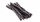A piece of licorice is 2/3 yard long.  If it is cut into 10 equal pieces, how long will each piece of licorice be?
• Divide 13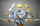Divide. Simplify your answer and write as an improper fraction or whole number. 14÷8/3
• The rope 2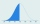The rope in the school gym is 10 ½ feet long. To make it easier to climb, Coach Hill tied a knot in the rope every ¾ foot. How many knots are in the rope?
• How many 16How many three-tenths are there in two and one-fourths?
• Fraction expressionWhich expression is equivalent to : minus 9 minus left parenthesis minus 4 start fraction 1 divided by 3 end fraction right parenthesis
• Cutting wireIf you cut a 3 ½ ft length wire into pieces that are 2 inches long, how many pieces of wire will you have?
• Evaluate expr with 2 varsIf a=6 and x=2, find the value of (2ax + 7x- 10) /(4ax-3a-2)
• Penny coinsOne 2p coin is 1/8 inch thick. A pile of 2p is 1 1/2 inch high. How many coin are there in the pile
• The length 7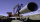The length of a water pipe is 7 m. How many ¼ m length can Jane cut from the pipe?
• Clothes 4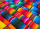If the cost of 16.5 metres of cloth is ₹2,062.50 then find the cost of 24.75 metres of clothes
• Evaluate mixed expressionsWhich of the following is equal to 4 and 2 over 3 divided by 3 and 1 over 2? A. 4 and 2 over 3 times 3 and 2 over 1 B. 14 over 3 times 2 over 7 C. 14 over 3 times 7 over 2 D. 42 over 3 times 2 over 31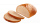There are 12 slices of bread and each person gets 3/4 of a slice of bread. How many people get bread?​
• Rewrite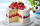Rewrite the following as division statement  if there are 8 cakes and each person get 1/12 of a cake how many people get cake?
• Quotient and productIf the quotient of [8/5 divided by 8/10] is added to the product of [8/14 x 7/12 x 3/8], what is the sum?
• The length 6The length of 12 pipes is 10 1/2 meters. (1) find the length of one pipe (2) also find the length of 7 pipes

Do you have an exciting math question or word problem that you can't solve? Ask a question or post a math problem, and we can try to solve it.

We will send a solution to your e-mail address. Solved examples are also published here. Please enter the e-mail correctly and check whether you don't have a full mailbox.

Please do not submit problems from current active competitions such as Mathematical Olympiad, correspondence seminars etc...

Need help to calculate sum, simplify or multiply fractions? Try our fraction calculator. Fraction Word Problems. Division Problems.